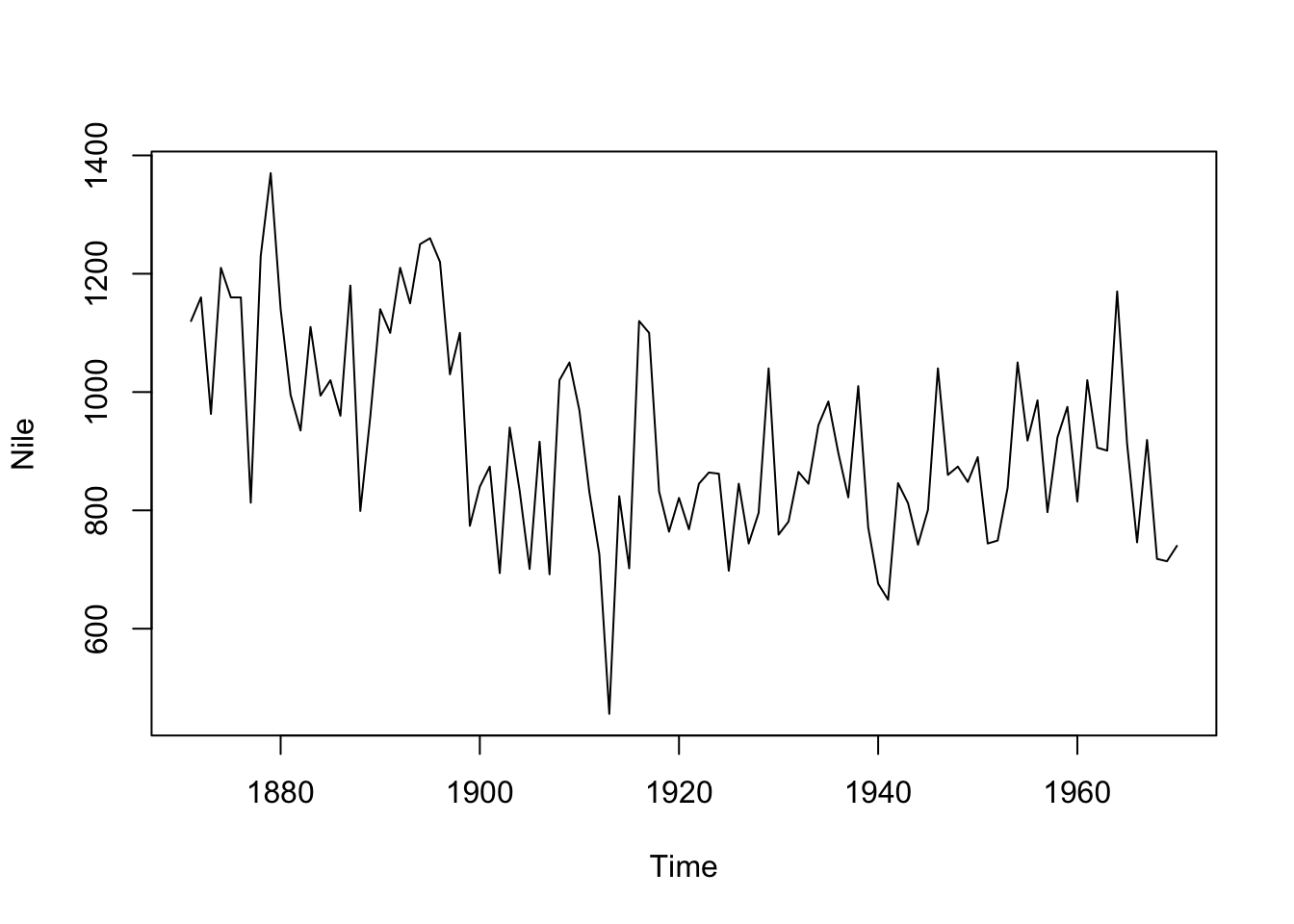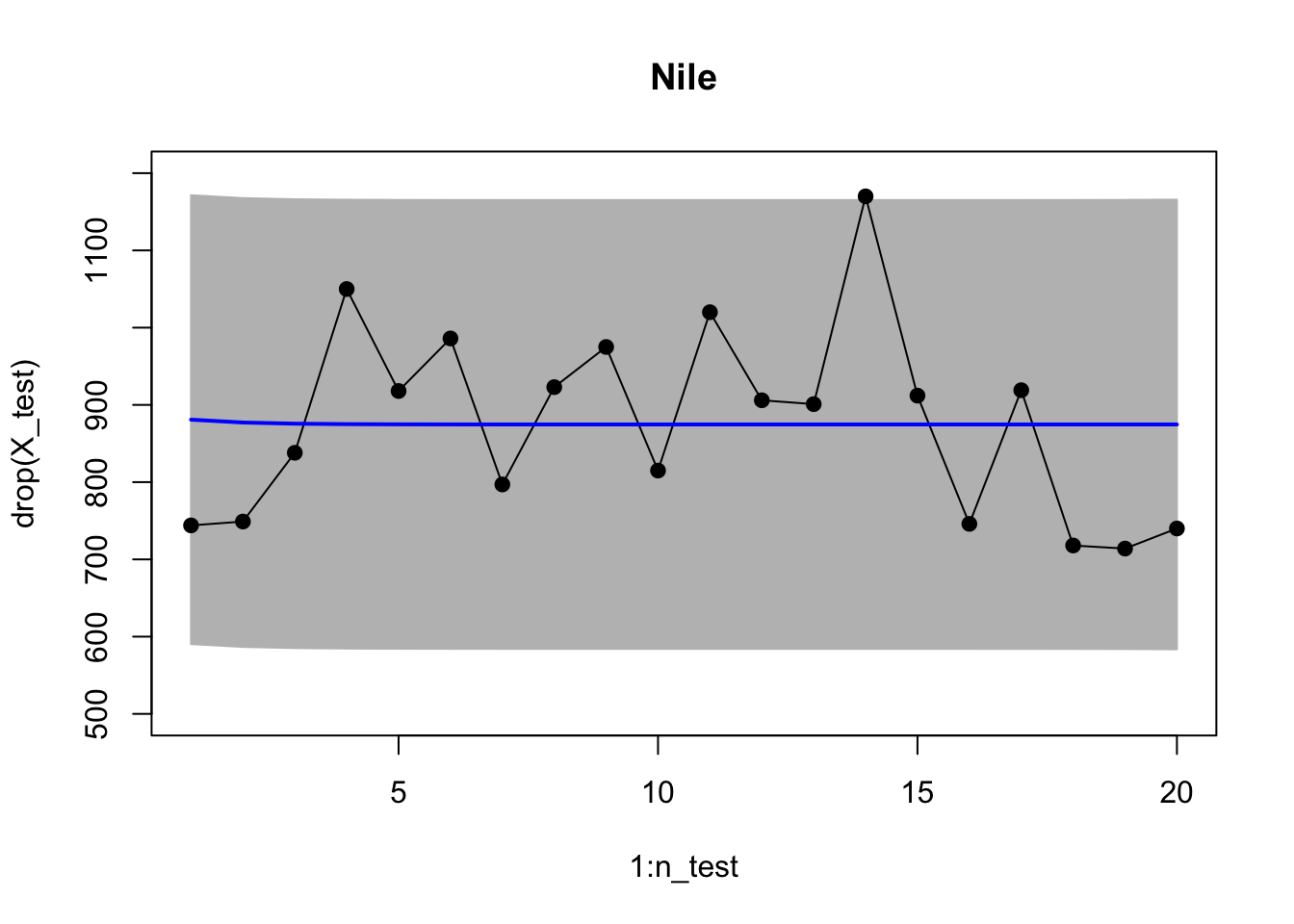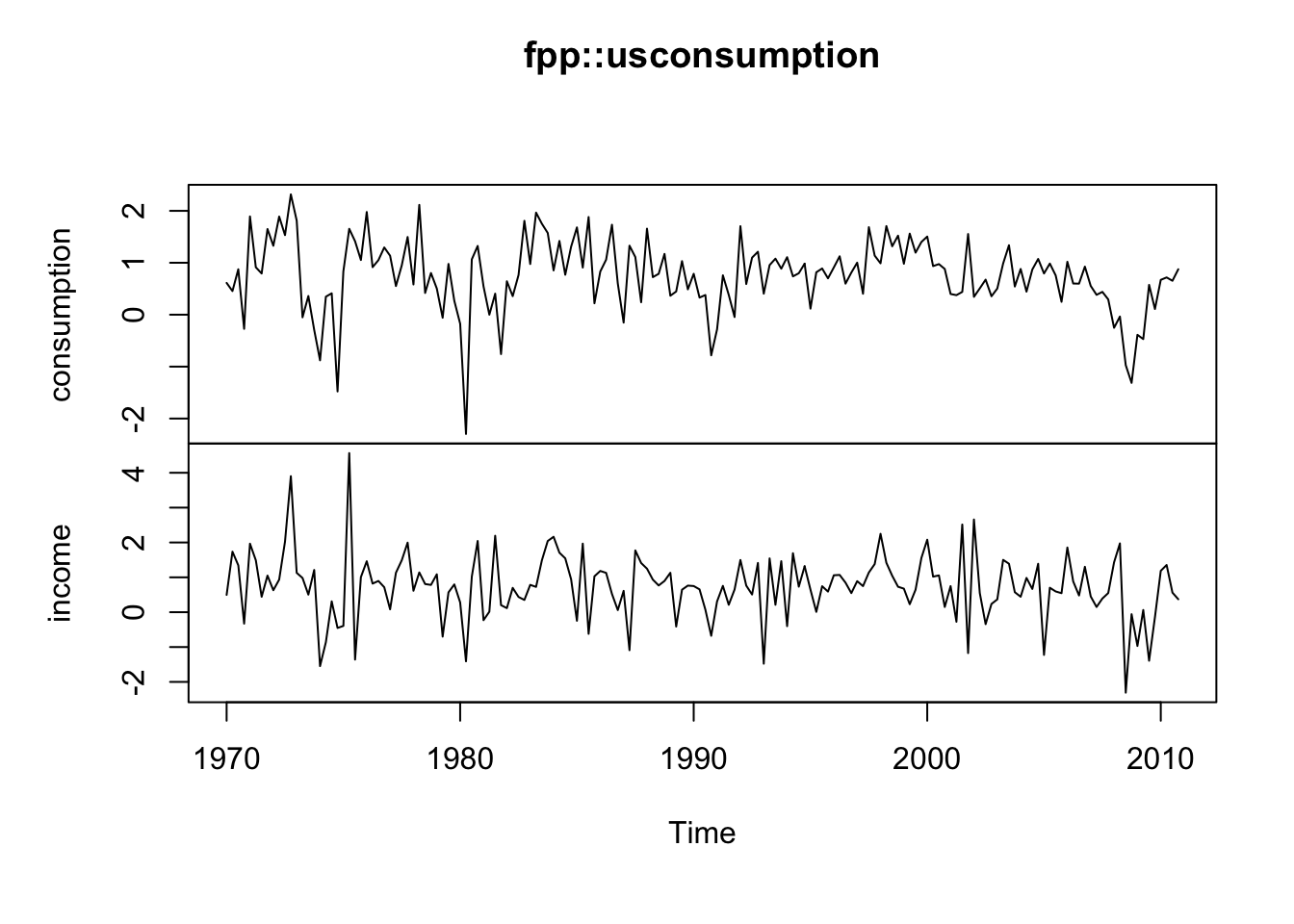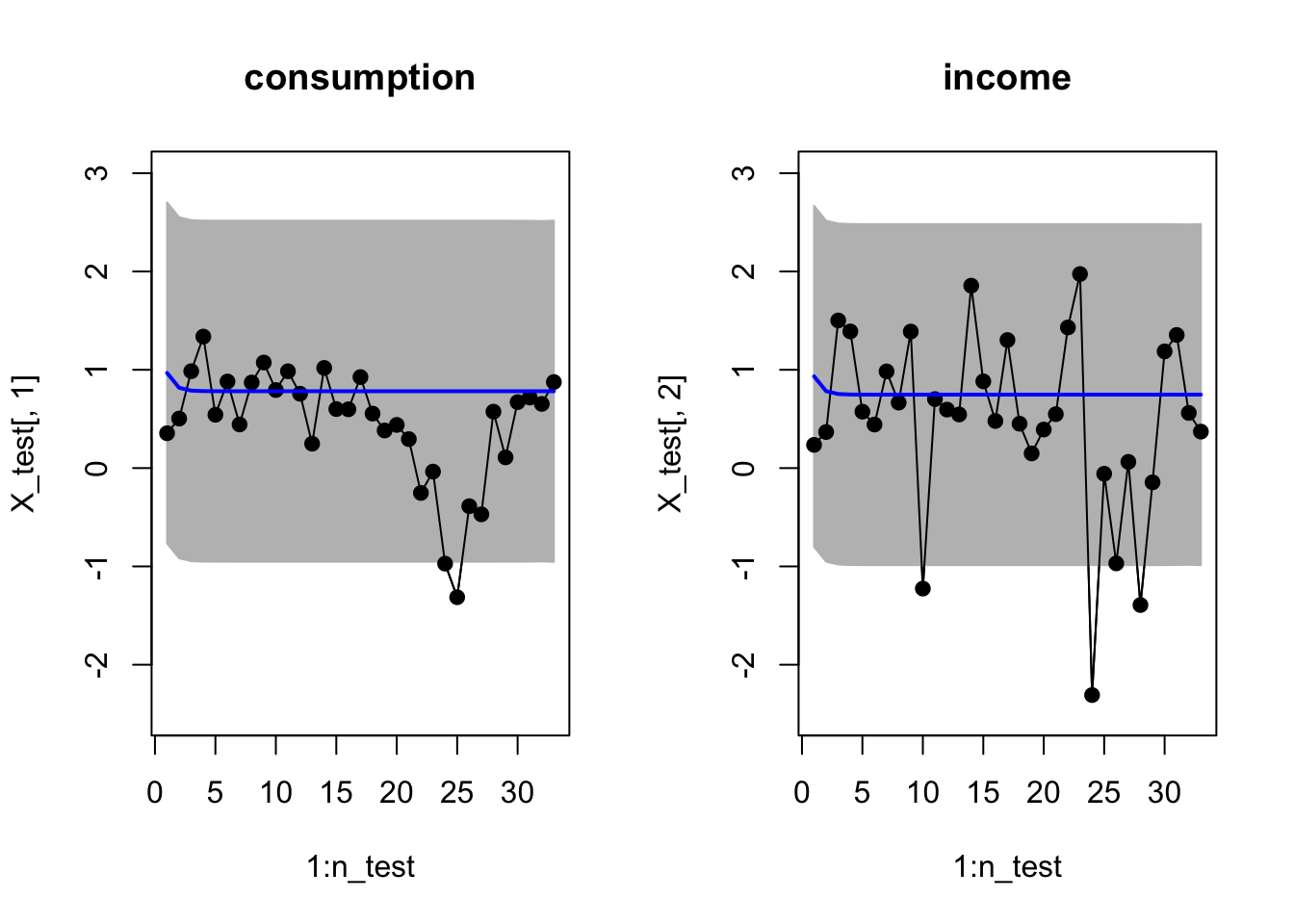This post is about Bayesian forecasting of univariate/multivariate time series in nnetsauce.

For each statistical/machine learning (ML) presented below, its default hyperparameters are used. A further tuning of their respective hyperparameters could, of course, result in a much better performance than what’s showcased here.

# 1 - univariate time series

The Nile dataset is used as univariate time series. It contains measurements of the annual flow of the river Nile at Aswan (formerly Assuan), 1871–1970, in 10^8 m^3, “with apparent changepoint near 1898” (Cobb(1978), Table 1, p.249).

library(datasets)
plot(Nile)Split dataset into training/testing sets:

X <- matrix(Nile, ncol=1)
index_train <- 1:floor(nrow(X)*0.8)
X_train <- matrix(X[index_train, ], ncol=1)
X_test <- matrix(X[-index_train, ], ncol=1)


sklearn’s BayesianRidge() is the workhorse here, for nnetsauce’s MTS. It could actually be any Bayesian ML model possessing methods fit and predict (there’s literally an infinity of possibilities here for class MTS).

obj <- nnetsauce::sklearn$linear_model$BayesianRidge()
print(obj$get_params())  Fit and predict using obj: fit_obj <- nnetsauce::MTS(obj = obj) fit_obj$fit(X_train)
preds <- fit_obj$predict(h = nrow(X_test), level=95L, return_std=TRUE)  95% credible intervals: n_test <- nrow(X_test) xx <- c(1:n_test, n_test:1) yy <- c(preds$lower, rev(preds$upper)) plot(1:n_test, drop(X_test), type='l', main="Nile", ylim = c(500, 1200)) polygon(xx, yy, col = "gray", border = "gray") points(1:n_test, drop(X_test), pch=19) lines(1:n_test, drop(X_test)) lines(1:n_test, drop(preds$mean), col="blue", lwd=2)# 2 - multivariate time series

The usconsumption dataset is used as an example of multivariate time series. It contains percentage changes in quarterly personal consumption expenditure and personal disposable income for the US, 1970 to 2010. (Federal Reserve Bank of St Louis. http://data.is/AnVtzB. http://data.is/wQPcjU.)

library(fpp)
plot(fpp::usconsumption)Split dataset into training/testing sets:

X <- as.matrix(fpp::usconsumption)
index_train <- 1:floor(nrow(X)*0.8)
X_train <- X[index_train, ]
X_test <- X[-index_train, ]


Fit and predict:

obj <- nnetsauce::sklearn$linear_model$BayesianRidge()
fit_obj2 <- nnetsauce::MTS(obj = obj)

fit_obj2$fit(X_train) preds <- fit_obj2$predict(h = nrow(X_test), level=95L,
return_std=TRUE) # standardize output+#plot against X_test



95% credible intervals:

n_test <- nrow(X_test)

xx <- c(1:n_test, n_test:1)
yy <- c(preds$lower[,1], rev(preds$upper[,1]))
yy2 <- c(preds$lower[,2], rev(preds$upper[,2]))

par(mfrow=c(1, 2))
# 95% credible intervals
plot(1:n_test, X_test[,1], type='l', ylim=c(-2.5, 3),
main="consumption")
polygon(xx, yy, col = "gray", border = "gray")
points(1:n_test, X_test[,1], pch=19)
lines(1:n_test, X_test[,1])
lines(1:n_test, preds$mean[,1], col="blue", lwd=2) plot(1:n_test, X_test[,2], type='l', ylim=c(-2.5, 3), main="income") polygon(xx, yy2, col = "gray", border = "gray") points(1:n_test, X_test[,2], pch=19) lines(1:n_test, X_test[,2]) lines(1:n_test, preds$mean[,2], col="blue", lwd=2)